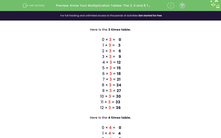# Know Your Multiplication Tables: The 3, 4 and 8 Times Tables

In this worksheet, students must recall multiplication facts from the 3, 4 and 8 times tables.Key stage:  KS 2

Curriculum topic:   Number: Multiplication and Division

Curriculum subtopic:   Use Multiplication/Division Facts (3, 4 and 8)

Popular topics:   Multiplication worksheets, Division worksheets, Times Table worksheets

Difficulty level:#### Worksheet Overview

In this activity, we will be practising our 3, 4, and 8 times table facts.

It's useful to know these facts by heart as it makes lots of maths calculations much quicker and easier.

However, if you don't yet know them all by heart here are a few tips:

Tip 1: Skip count to find the right multiple.

If you want to know 4 × 3, simply count up in 3s until you reach the 4th multiple: 3, 6, 9, 12.

Tip 2: Use a known fact.

If you want to know 9 × 4 and aren't sure of the answer, you could count back from an easier fact such as 10 × 4.

10 × 4 = 40

9 × 4 is 4 less than 40.

9 × 4 = 36

Tip 3: Use facts from the 4 times table to find multiples of 8.

If you know your 4 times table facts, you can simply double the multiple to find an 8 times table fact.

4 × 3 = 12

8 × 3 = 24

Can you see that 24 is double 12?

Now that you've picked up a few tips, you're ready to try the questions. Good luck.

### What is EdPlace?

We're your National Curriculum aligned online education content provider helping each child succeed in English, maths and science from year 1 to GCSE. With an EdPlace account you’ll be able to track and measure progress, helping each child achieve their best. We build confidence and attainment by personalising each child’s learning at a level that suits them.

Get started